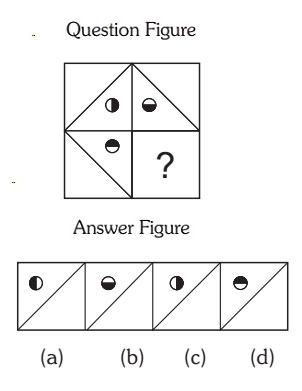#### Completion Of Incomplete Pattern

Direction: In each of the following questions, find out which of the answer figures (a), (b), (c) and (d) completes the figure?

1. Select the correct figure from the Answer Figure that would complete the Question Figure.1. Option (a) in Answer Figure.
2. Option (b) in Answer Figure.
3. Option (c) in Answer Figure.
4. Option (d) in Answer Figure.
##### Correct Option: A

figure inside the box moves 90 degree in clockwise direction each time. So if you move the 3rd box figure in clockwise direction with 90 degree, we will get the option (a) is right figure to complete.